Published on

# Algorithms Explained Fast | DSA Series | Part 3 of 3 | Blog Post 3

Author
•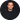Name
Robert Kirby
Title
Lead Full Stack Developer at Y-Squared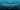ALGORITHMS EXPLAINED FAST

Data Structures and Algorithms Series | Part 3 of 3 | Blog Post 3

#### 3 Sorting Algorithms

• 3.1 Bubble Sort
• 3.2 Selection Sort
• 3.3 Insertion Sort
• 3.4 Merge Sort
• 3.5 Quick Sort

#### 4 Searching Algorithms

• 4.1 Linear Search (Lists)
• 4.2 Binary Search (Sorted lists)
• 4.3 Naive Search (String/Pattern Matching)
• 4.5 BFS: Breadth-First Search & DFS: Depth-First Search (Trees & Graphs)
##### 5 Contact, Links & GitHub Repo

*GitHub Repo: Link located at the end of this post*

## 1. Introduction

Welcome to the third and final part of our series on Data Structures and Algorithms: Algorithms Explained Fast!

What are algorithms? In computer science, an algorithm is a list of instructions, used to solve problems or perform tasks.

During this post we will work through some of the most popular algorithms out there with easy to understand explanations, diagrams and code examples.

Keeping to the title of this post we will hit these algorithms fast:

• There will be a diagram for each to help you visualize the process.
• We will then go over a short language independent explanation of each algorithm.
• We will then look at code examples (written in JavaScript).
• We will not be doing full code walkthroughs in this blog post as the examples are for reference, But I will leave notes on some of the examples.
• We will also reference Big-O throughout this post !

If you would like to read the other parts of this blog series here are the links:

2 Recursion

Recursion is not an algorithm but a concept; it is used across searching and sorting algorithms so we must recursion before moving forward. Recursion is when a function or algorithm calls itself one or more times until a specified condition is met. Recursion is good when tasks have repeated sub tasks to do. For example when searching a file tree on a computer where folders have subfolders and so on.

Recursive functions must have a base case to stop the function. Otherwise you will get an infinite loop.

Recursion Example

``````let count = 1; // Initial count

function recursiveFunction() {
console.log(`Recursive \${count}`);
if (count === 10) return console.log("Stopped"); // Base Case or Stop point: Condition if true stop function
count++; // if false, increment the count
recursiveFunction(); // recursive call to itself - When it stops it will return "Stopped"
}

recursiveFunction();
``````

Stack Overflow:

The example below has no base case or condition to stop the recursions resulting in an infinite loop.

This sort of function would normally result in crashing the program it’s running on, but most browsers and IDE’s for example have built in safeguards where the stack size is limited and will just return an error in the console like this instead “RangeError: the maximum stack size will be exceeded”; this is called stack overflow.

Infinite Loop Recursive Example:

``````function recursiveFunction() {
console.log("infinite loop");
recursiveFn();
}

recursiveFn();
``````

Recursion vs Iteration:

Anything you can do with a recursion can be done with a loop(Iteratively), so why would we use recursion? It really depends on the function or algorithm.

Recursion can have better readability and less code, but it can also have a large stack size costing in memory. Loops on the other hand can be more efficient but less readable.

Recursion is good when we have a data structure where we might not know how deep the data set is, you might encounter this on a tree for example.

Note:There is a feature called tail call optimization that is available in most languages, including Javascript; it allows us to solve the large stack size issue with recursion. If you want to learn more about this it is only fitting that we turn to Stack Overflow for the answer: https://stackoverflow.com/questions/310974/what-is-tail-call-optimization

## 3 Sorting Algorithms

A sorting algorithm is used to sort or rearrange a list of elements.

#### 3.1 Bubble Sort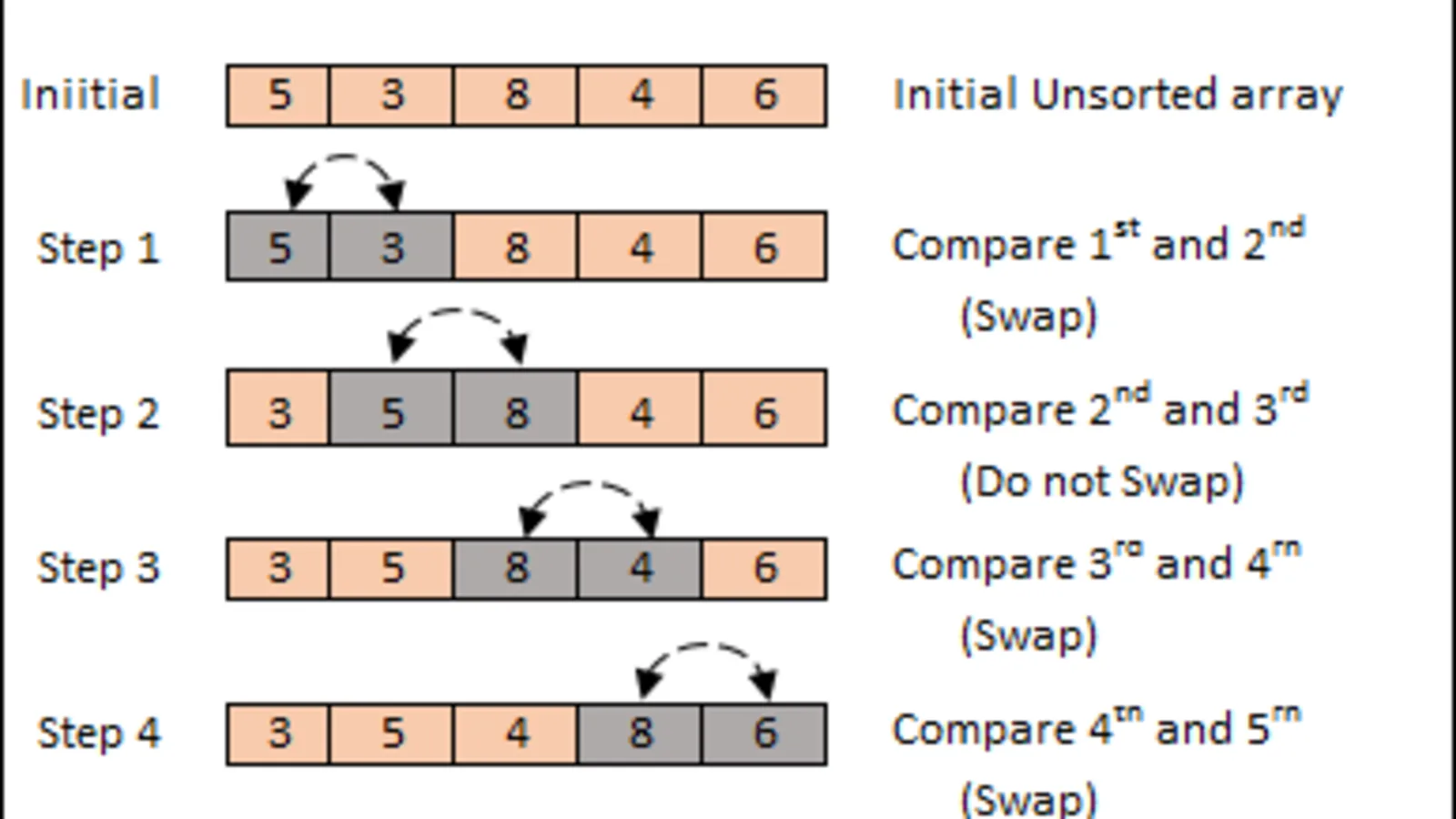chart source:https://dev.to/buurzx/buble-sort-4jkc

Explanation:

Bubble sort is a simple sorting algorithm that works by comparing adjacent elements and swapping them if they are in the wrong order, the larger numbers buble to the top! It may take multiple passes to complete the sorting process. Bubble sort is not suitable for large data sets as it is inefficient with time complexity.

Bubble Sort Example:

``````const array = [1, 4, 2, 300, 2002, 92];

function bubbleSort(array) {
for (let i = 0; i < array.length; i++) {
for (j = 0; j < array.length - i - 1; j++) {
if (array[j] > array[j + 1]) {
const temp = array[j];
array[j] = array[j + 1];
array[j + 1] = temp;
// Can also use this instead of temp variable [array[j], array[j + 1]] = [array[j + 1], array[j]];
}
}
}
return array;
}

console.log(bubbleSort(array));
``````

Notes:We use - i - 1 on the inner for loop because after each pass the largest numbers go to the end( or bubble to the top) so there is no need to keep iterating over them so we decrease the length to improve efficiency.

Time & Space Complexity:

• Worst case time - O(n^2) - Quadratic
• Best case time - O(n) - Linear
• Space Complexity - O(1) - Constant

#### 3.2 Selection Sort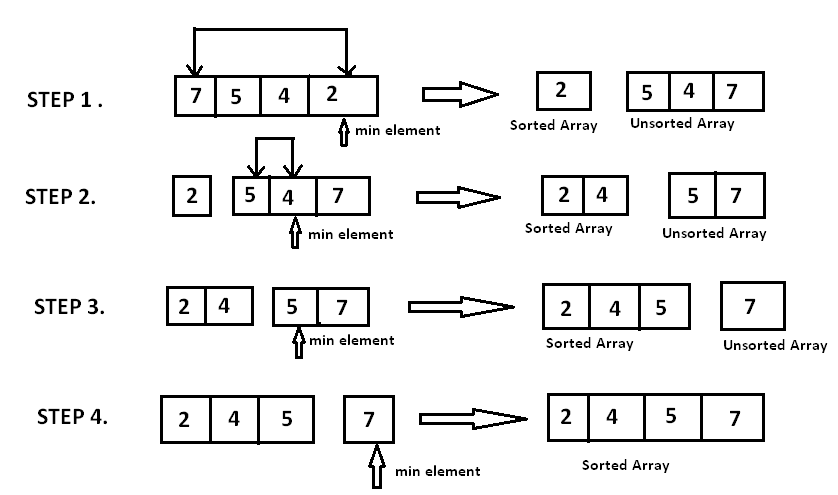Explanation:

Selection Sort is used to sort a list of elements by repeatedly finding the next minimum element. Every time it finds an element it makes another pass until it is completed. Like bubble sort this algorithm is also not suitable for large data sets.

The algorithm keeps two sub arrays in the given array:

• Sorted sub array
• Unsorted sub array

With every pass it takes an element from the unsorted array and moves it to the sorted array until the task is completed.

Selection Sort Example:

``````function selectionSort(array) {
for (let i = 0; i < array.length - 1; i++) {
let minIndex = i;
for (let j = i; j < array.length; j++) {
if (array[j] < array[minIndex]) {
}
}
const temp = array[i];
array[i] = array[minIndex];
array[minIndex] = temp;
//  [array[i], array[minIndex]] = [array[minIndex], array[i]]; // Can also use this code instead
}
return array;
}

const selArray = [1, 4, 2, 300, 2002, 92];

console.log(selectionSort(selArray));
``````

Notes:

• We use array.length - 1 because the last element will always be the largest and there won't be anything to compare it to.
• We let j = i because as we go through our iterations, the elements at the beginning become sorted and there is no need to go over them again, this improves efficiency and is also how the two sub-arrays as mentioned above are created.

Time & Space Complexity:

• Worst case time - O(n^2) Quadratic
• Best case time - O(n^2) Quadratic
• Space Complexity - O(1) Constant

#### 3.3 Insertion Sort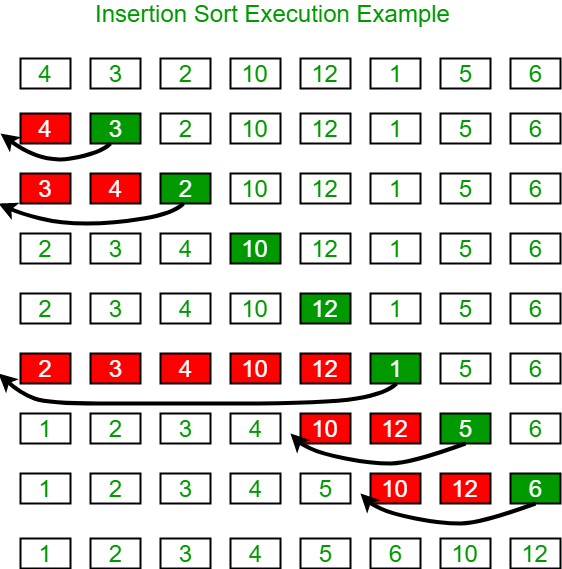Chart Source:https://www.geeksforgeeks.org/insertion-sort/

Explanation:

Insertion sort is a simple sorting algorithm. It works by taking an unsorted list and putting the elements into a sorted subsection of the list one at a time. Think of the list as broken into two sections unsorted and sorted; it will make multiple passes over the list until this is completed.

Insertion Sort Example:

``````function insertionSort(array) {
for (let i = 1; i < array.length; i++) {
for (let j = i; j > 0; j--) {
if (array[j] < array[j - 1]) {
[array[j], array[j - 1]] = [array[j - 1], array[j]];
} else {
break;
}
}
}
return array;
}

const inserArray = [69, 72, 1, 4, 2, 300, 2002, 92, 45, 120];

console.log(insertionSort(inserArray));
``````

Notes:

• Insertion sort is often compared to having playing cards in your hand.
• It is virtually sorted into a sorted and unsorted array. In the example we no longer check the elements on the left hand side of the array once they have been compared and swapped, to improve efficiency.

Time & Space Complexity:

• Worst case time - O(n^2) Quadratic
• Best case time - O(n) Linear
• Space Complexity - O(1) Constant

#### 3.4 Merge Sort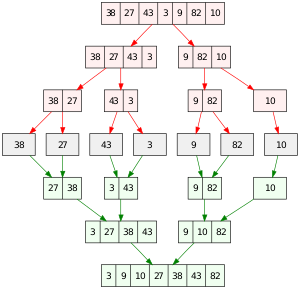Chart source:

Explanation:

Merge sort is a recursive algorithm that uses the divide and conquer method. It divides the list in half (This can happen more than once - recursively); it then sorts each part as there own smaller lists then merges the lists back together in a sorted manner.

Merge Sort Example:

``````// Helper function - left and right are sorted
function merge(leftArray, rightArray) {
const output = [];
let leftIndex = 0;
let rightIndex = 0;

while (leftIndex < leftArray.length && rightIndex < rightArray.length) {
const leftElement = leftArray[leftIndex];
const rightElement = rightArray[rightIndex];
if (leftElement < rightElement) {
output.push(leftElement);
leftIndex++;
} else {
output.push(rightElement);
rightIndex++;
}
}
return [
...output,
...leftArray.slice(leftIndex),
...rightArray.slice(rightIndex),
];
}

// Merge Sort Function
function mergeSort(array) {
if (array.length <= 1) {
return array;
}
const middleIndex = Math.floor(array.length / 2);
const leftArray = array.slice(0, middleIndex);
const rightArray = array.slice(middleIndex);
return merge(mergeSort(leftArray), mergeSort(rightArray));
}

const merArray = [69, 72, 1, 4, 2, 300, 2002, 92, 82, 82, 4000];
console.log(mergeSort(merArray));
``````

Time & Space Complexity:

• Worst case time - O(n log (n)) Log Linear
• Best case time - Ω(n log(n)) Log Linear - Lower Bound
• Space Complexity - O(n) Linear

Not: - Ω = Omega -> Big O represents the upper bound, Big Omega represents the lower bound.

#### 3.5 Quick Sort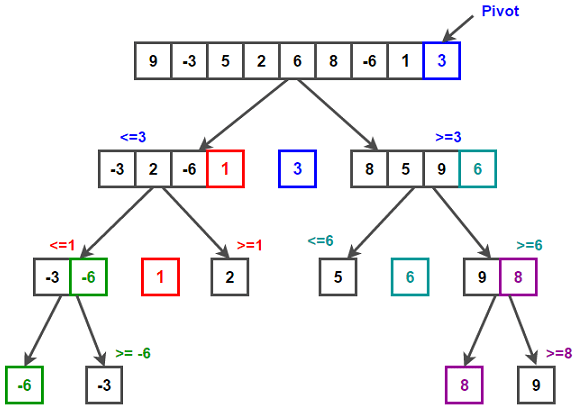Explanation:

Quick sort is another divide and conquer style algorithm. It works by picking a pivot element and partitioning the list into two parts (The pivot element is usually the first or last element); one side with elements of a lesser value and the other side with elements of a greater value, this is done multiple times in a recursive manner until the list is sorted.

Quick Sort Example:

``````function quickSort(array) {
if (array.length == 1) {
return array;
}
const pivot = array[array.length - 1];
const leftArray = [];
const rightArray = [];
for (let i = 0; i < array.length - 1; i++) {
if (array[i] < pivot) {
leftArray.push(array[i]);
} else {
rightArray.push(array[i]);
}
}

if (leftArray.length > 0 && rightArray.length > 0) {
return [...quickSort(leftArray), pivot, ...quickSort(rightArray)];
} else if (leftArray.length > 0) {
return [...quickSort(leftArray), pivot];
} else {
return [pivot, ...quickSort(rightArray)];
}
}

const quiArray = [69, 600, 72, 1, 4, 670, 82, 2, 300, 2002, 92];
console.log(quickSort(quiArray));
``````

Time & Space Complexity:

• Worst case time - O(n^2) Quadratic
• Best case time - Ω(n log(n)) Log Linear - Lower Bound
• Space Complexity - O(log n) Logarithmic

## 4 Searching Algorithms

Searching algorithms are used to check for or retrieve an element from a data structure. Unlike the sorting algorithms which are used to arrange a list of Items into a specific order, the Big-O of searching algorithms depend on the data structures they are used with.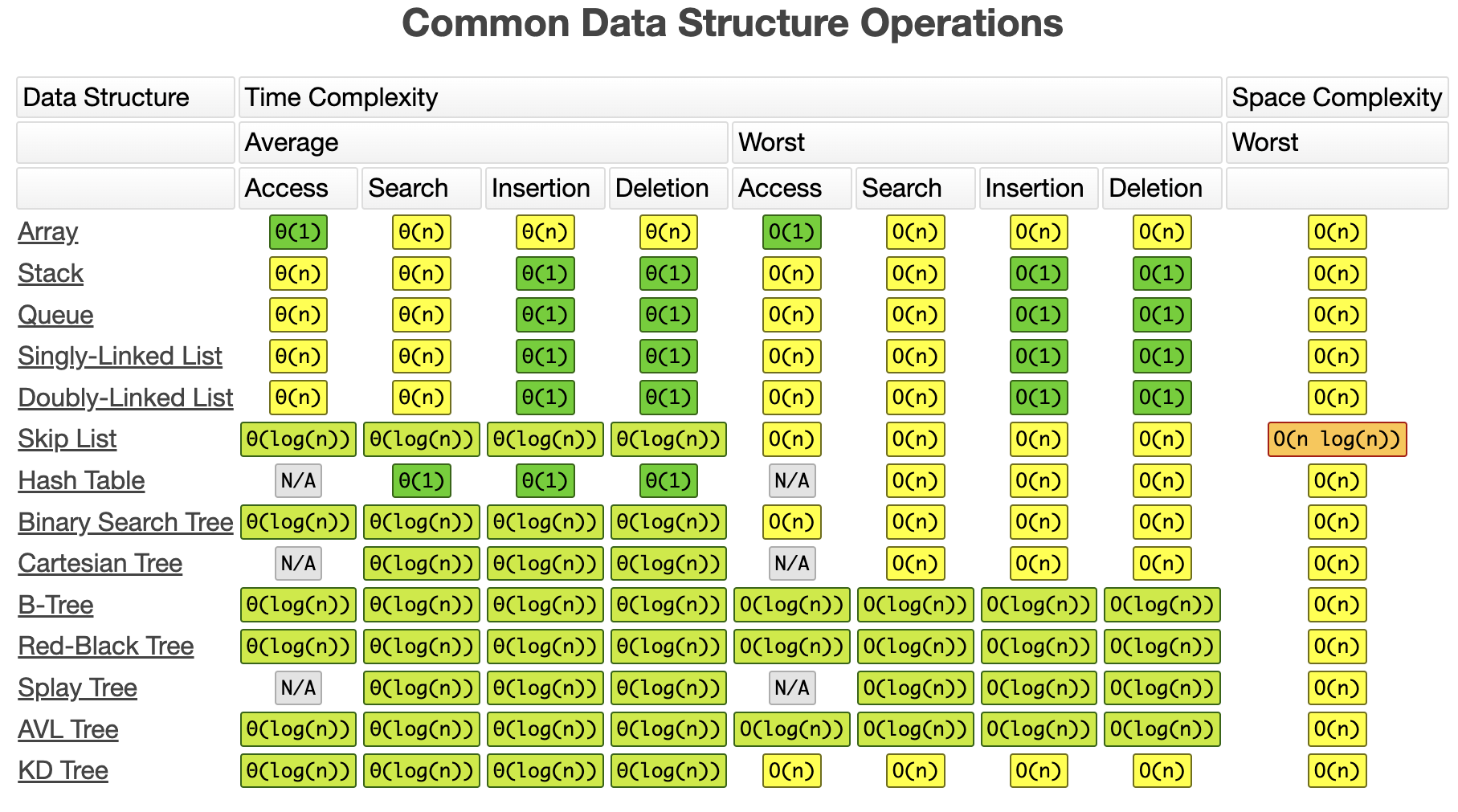Screen shot taking from:https://www.bigocheatsheet.com/

#### 4.1 Linear Search (Lists)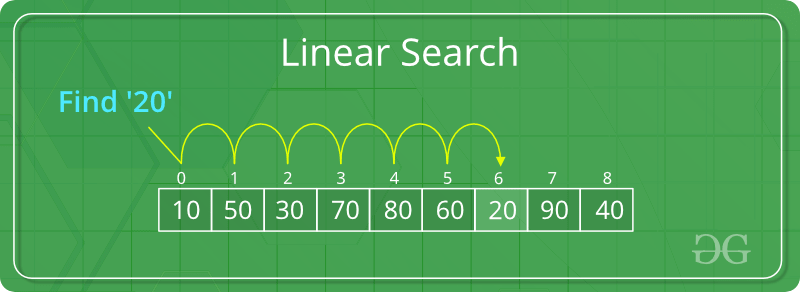Chart source:https://www.geeksforgeeks.org/linear-search/

Explanation:

Linear search is a simple searching algorithm that takes a list as an input and loops through the list one by one until the desired element is found.

We can use the Iterative method and a recursive method to carry out linear search.

On most data structures, linear search will have a time complexity of 0(n) - Linear time.

Linear Search Example:

``````function linearSearch(array, item) {
for (var i = 0; i < array.length; i++) {
if (array[i] === item) {
return console.log(`LINEAR SEARCH: \${item} found in list at index \${i}!`);
}
}
}

const lsArray = [69, 600, 72, 1, 4, 670, 82, 2, 300, 2002, 92];
linearSearch(lsArray, 670);
``````

#### 4.2 Binary Search (Sorted lists)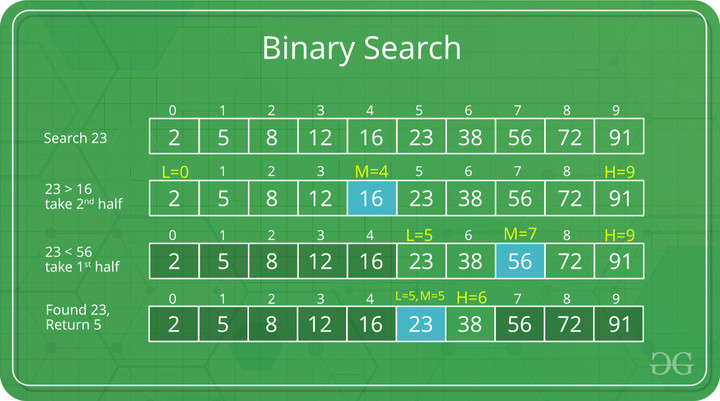Chart source:https://www.geeksforgeeks.org/binary-search/?ref=lbp

Explanation:

Binary search is a searching algorithmused on sorted lists, it uses the divide and conquer method by repeatedly dividing the search interval in half until it finds the desired element.

When used on a sorted array it reduces in time complexity as the algorithm progresses through the dataset leading to a time complexity of O(log n) - Logarithmic time.

Like linear search we can use both an iterative method & recursive method to carry it out.

Binary Search Example:

``````function binarySearch(sortedArray, key) {
let start = 0;
let end = sortedArray.length - 1;
while (start <= end) {
let middle = Math.floor((start + end) / 2);
if (sortedArray[middle] === key) {
return console.log(`BINARY SEARCH: key(\${key}) found in list!`);
} else if (sortedArray[middle] < key) {
start = middle + 1;
} else {
end = middle - 1;
}
}
}

let sa = [1, 2, 2, 3, 4, 5, 6, 7, 8];

binarySearch(sa, 5);
``````

#### 4.3 Naive Search (String/Pattern Matching)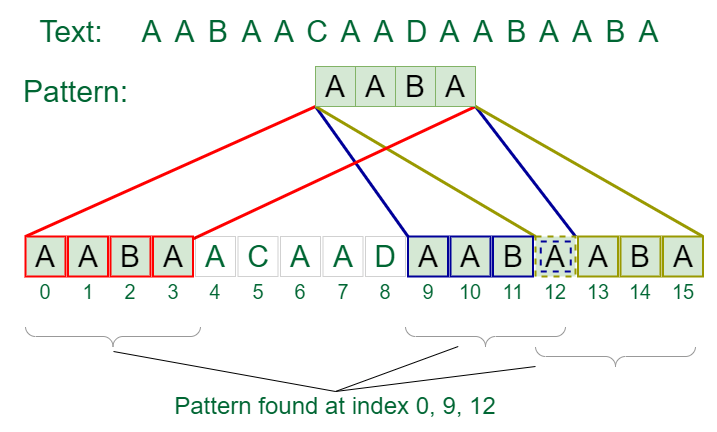Explanation:

Naive search is a pattern searching algorithm and can also be called a string searching algorithm. There are usually two inputs. The first is the input you want to search (A paragraph of text for example), the second is the pattern you want to search for within the first input.

Naive Search Example:

``````function naiveSearch(string, pattern) {
let count = 0;
for (let i = 0; i < string.length; i++) {
for (let j = 0; j < pattern.length; j++) {
if (pattern[j] !== string[i + j]) break;
if (j === pattern.length - 1) count++;
}
}
if (count === 0) {
} else {
return `The pattern "\${pattern}" was found \${count} times in the string input!`;
}
}

console.log(naiveSearch("codexxxsdxcsciocodevndedsvsddnxccode", "code"));
``````

#### 4.4 BFS: Breadth-First Search & DFS: Depth-First Search (Trees & Graphs)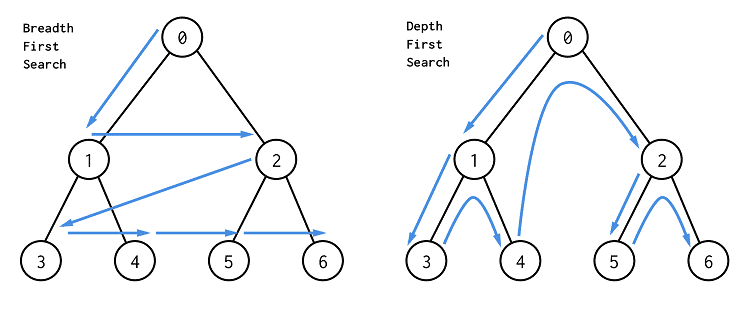Explanation:

Breadth First Search and Depth First Search are searching/traversal algorithms used on graph and tree data structures. We will focus on trees in the below example and explanations.

Breadth first search starts at the root node working its way down each level checking sibling nodes left to right before moving onto their children on the next level. Usually a queue data structure is used to keep track of the child nodes that were encountered but not yet explored

Depth first search works by traversing the full dept of a branch before proceeding onto the next branch. There are a few different methods, they are In-Order, Pre-Order and Post-Order. Usually a stack is used to keep track of the nodes traversed along a branch which helps with the backtracking of the graph.

We will now explore some examples. If you would like an in depth comparison on the difference between BFS and DFS I recommend reading this section on Geeks for Geeks: https://www.geeksforgeeks.org/difference-between-bfs-and dfs/#:~:text=BFS%20is%20a%20traversal%20approach,with%20no%20unvisited%20nearby%20nodes.

This next example is sample code taken from Andrei Neagoie @aneagoie on Replit with some minor modifications.

BFS & DFS Examples:

``````// Node
class Node {
constructor(value) {
this.left = null;
this.right = null;
this.value = value;
}
}

// Binary Search Tree Example - We've included the insert and lookup methods.
// BFS and DFS methods are also included.

class BinarySearchTree {
constructor() {
this.root = null;
}
//  Insert Method
insert(value) {
const newNode = new Node(value);
if (this.root === null) {
this.root = newNode;
} else {
let currentNode = this.root;
while (true) {
if (value < currentNode.value) {
if (!currentNode.left) {
currentNode.left = newNode;
return this;
}
currentNode = currentNode.left;
} else {
if (!currentNode.right) {
currentNode.right = newNode;
return this;
}
currentNode = currentNode.right;
}
}
}
}
// Lookup Method
lookup(value) {
if (!this.root) {
return false;
}
let currentNode = this.root;
while (currentNode) {
if (value < currentNode.value) {
currentNode = currentNode.left;
} else if (value > currentNode.value) {
currentNode = currentNode.right;
} else if (currentNode.value === value) {
return currentNode;
}
}
return null;
}
// BFS Method
breathFirstSearch() {
let currentNode = this.root;
let list = [];
let queue = [];
queue.push(currentNode);
while (queue.length > 0) {
currentNode = queue.shift();
list.push(currentNode.value);
if (currentNode.left) {
queue.push(currentNode.left);
}
if (currentNode.right) {
queue.push(currentNode.right);
}
}
}
// DFS Methods
DFSInOrder() {
return traverseInOrder(this.root, []);
}
DFSPostOrder() {
return traversePostOrder(this.root, []);
}
DFSPreOrder() {
return traversePreOrder(this.root, []);
}
}

// In-Order
function traverseInOrder(node, list) {
// console.log(node.value);
if (node.left) {
traverseInOrder(node.left, list);
}
list.push(node.value);
if (node.right) {
traverseInOrder(node.right, list);
}
return console.log(`DFS: InOrder: \${list}`);
}

// Pre-Order
function traversePreOrder(node, list) {
// console.log(node.value);
list.push(node.value);
if (node.left) {
traversePreOrder(node.left, list);
}
if (node.right) {
traversePreOrder(node.right, list);
}
return console.log(`DFS: PreOrder: \${list}`);
}

// Post-Order
function traversePostOrder(node, list) {
// console.log(node.value);
if (node.left) {
traversePostOrder(node.left, list);
}
if (node.right) {
traversePostOrder(node.right, list);
}
list.push(node.value);
return console.log(`DFS: PostOrder: \${list}`);
}

// New Tree & Inserting Data
const tree = new BinarySearchTree();
tree.insert(9);
tree.insert(4);
tree.insert(6);
tree.insert(20);
tree.insert(170);
tree.insert(15);
tree.insert(1);

// Call the methods
tree.breathFirstSearch();
tree.DFSInOrder();
tree.DFSPreOrder();
tree.DFSPostOrder();

// How the tree would look
//     9
//  4     20
//1  6  15  170

//Outputs
// BFS: Breadth First Search: 9,4,20,1,6,15,170
// Breadth First Search = Root, level 1: left to right, level 2: left to right

// DFS: InOrder: 1,4,6,9,15,20,170 // Inorder = Left, Root, Right
// DFS: PreOrder: 9,4,1,6,20,15,170 // Preorder = Root, Left, Righ
// DFS: PostOrder: 1,6,4,15,170,20,9 // Post Order = Left, Right, Root
``````

## 5 Contact, Links & GitHub Repo

Please fill out the form on https://y-squared.com/contact or email us at team@y-squared.com.

The Author:This post was written by Robert from Y-Squared:

Blog Site:

https://y-squared.blog/